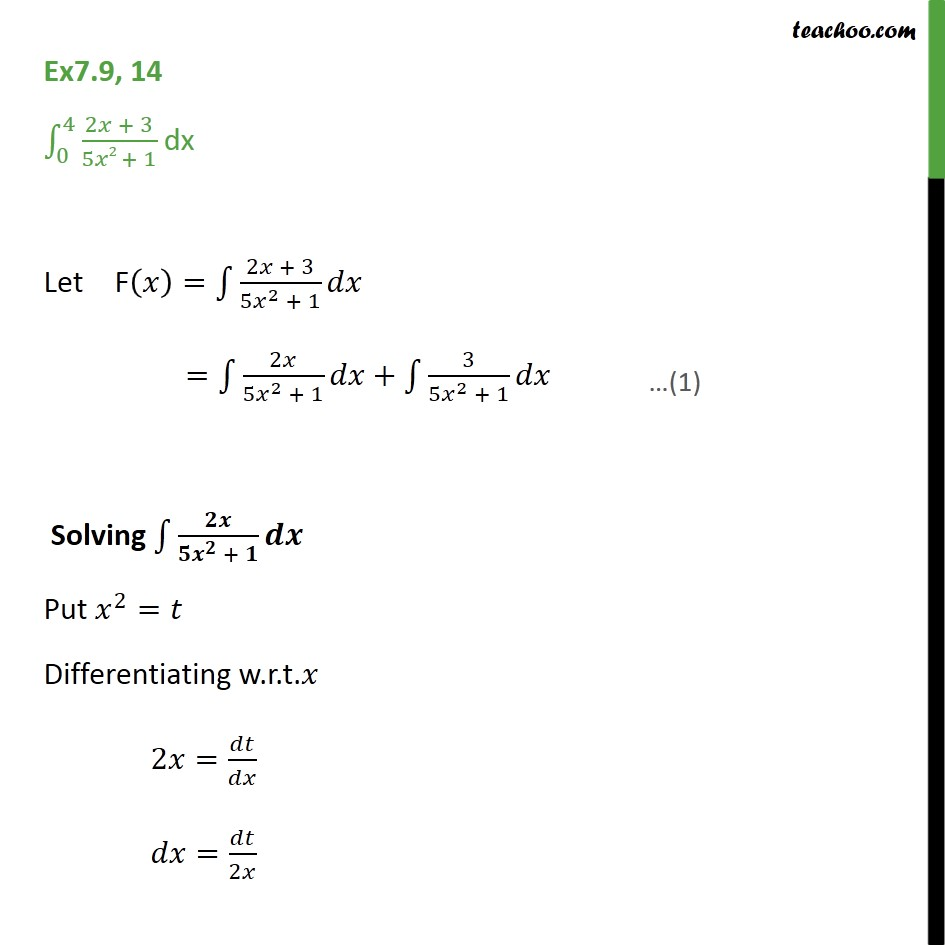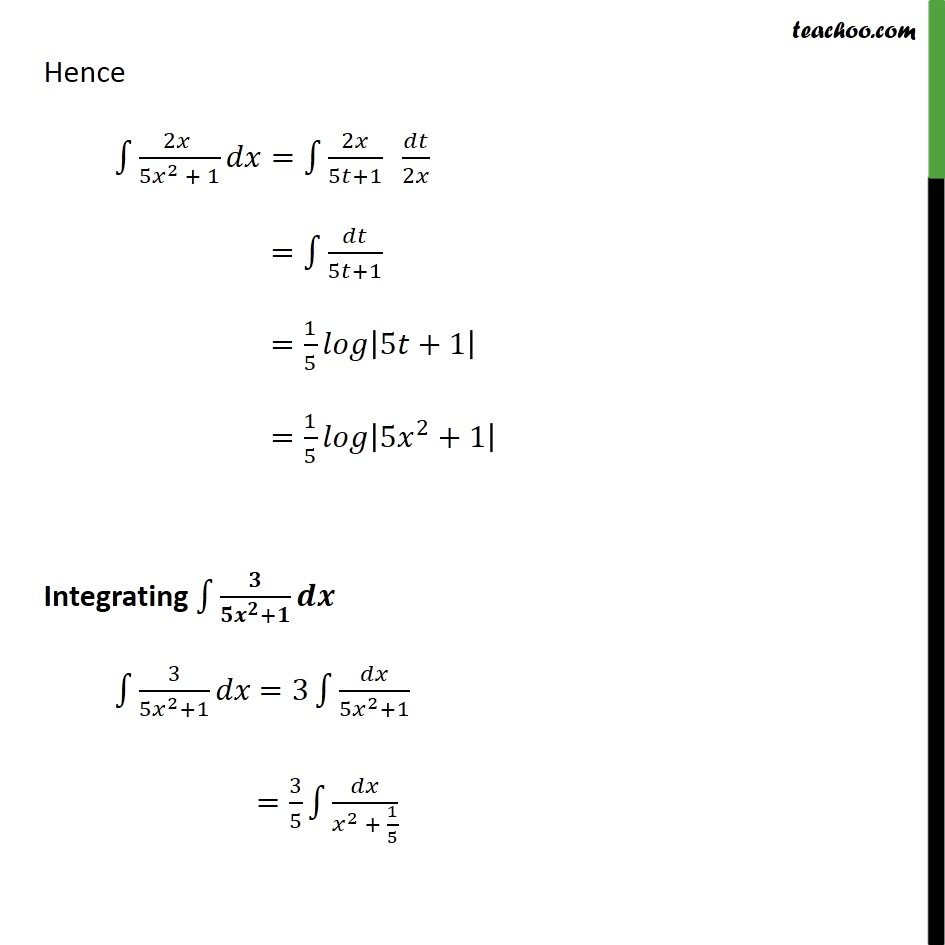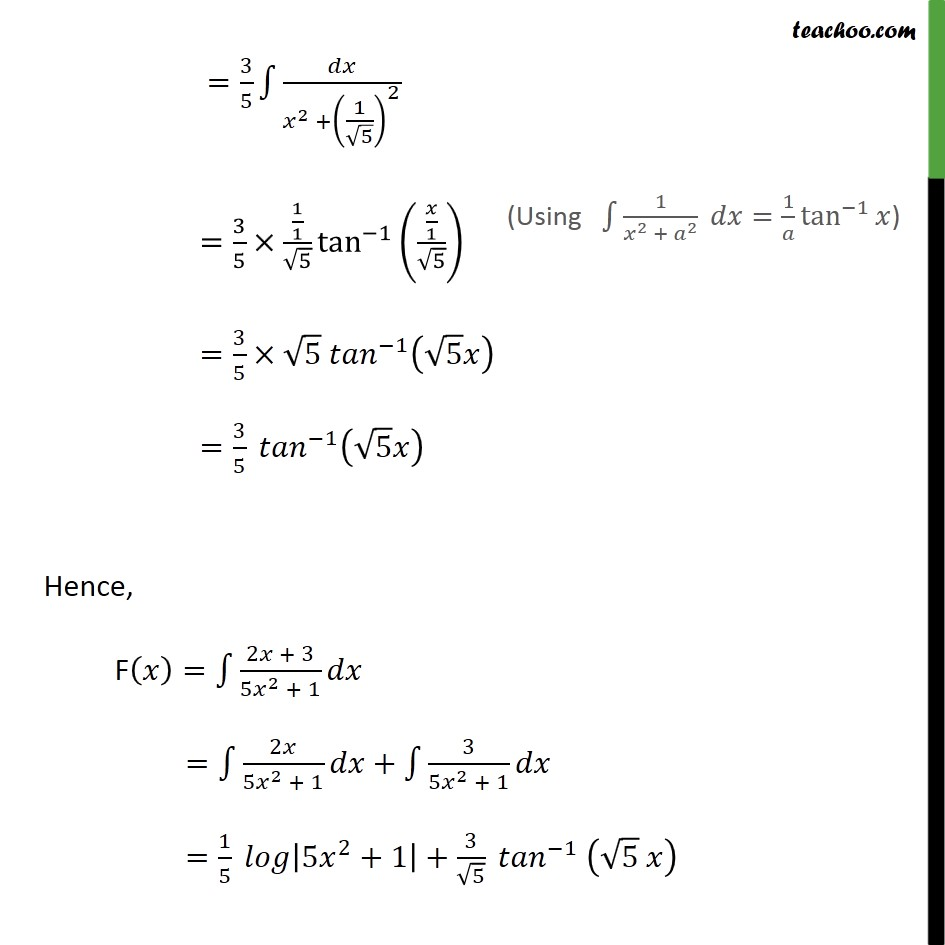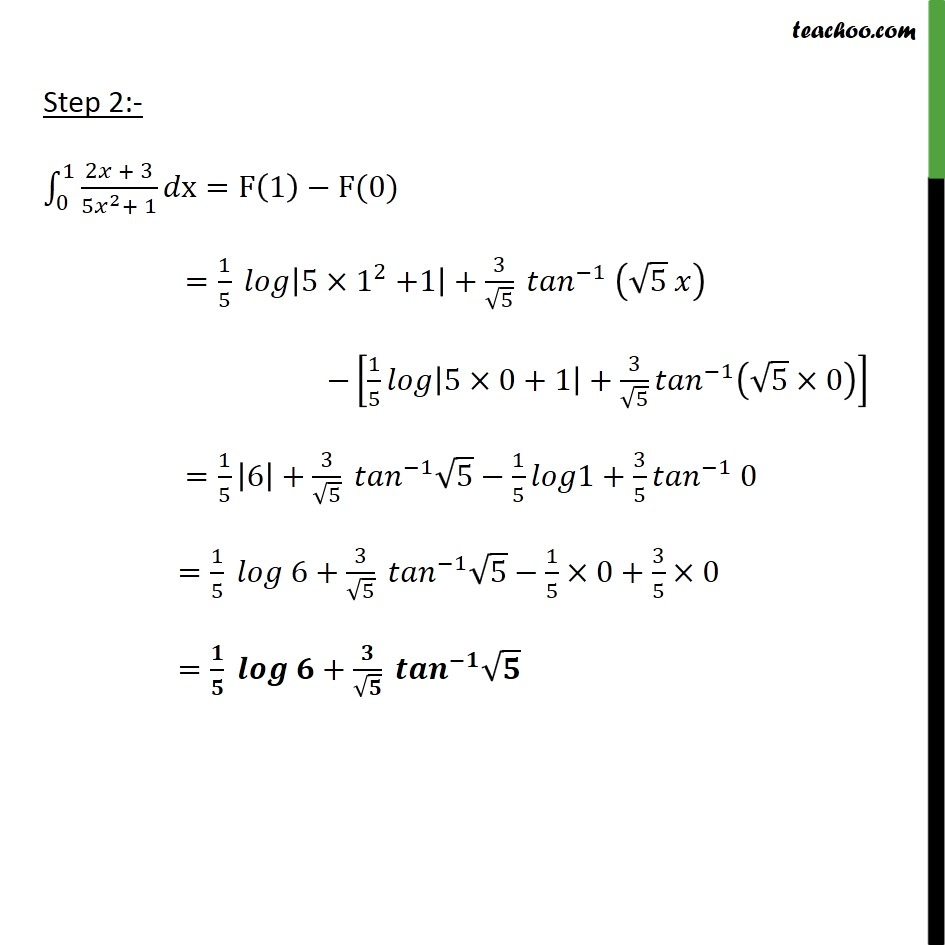Integration Full Chapter Explained - Integration Class 12 - Everything you need1. Chapter 7 Class 12 Integrals
2. Concept wise
3. Definite Integration - By Formulae

Transcript

Ex7.9, 14 _0^4 (2 + 3)/(5 2 + 1) dx _0^1 (2 + 3)/( 5 ^2+ 1) x=F(1) F(0) =1/5 |5 1 ^2+1|+3/ 5 ^( 1) ( 5 ) [1/5 |5 0+1|+3/ 5 ^( 1) ( 5 0)] =1/5 |6|+3/ 5 ^( 1) 5 1/5 1+3/5 ^( 1) 0 =1/5 6+3/ 5 ^( 1) 5 1/5 0+3/5 0 = / + / ^( )

Definite Integration - By Formulae

About the AuthorDavneet Singh
Davneet Singh is a graduate from Indian Institute of Technology, Kanpur. He has been teaching from the past 9 years. He provides courses for Maths and Science at Teachoo.